# Lookout tower

Calculate the height of a lookout tower forming a shadow of 36 m if at the same time a column 2.5 m high has a shadow of 1.5 m.

h =  60 m

### Step-by-step explanation: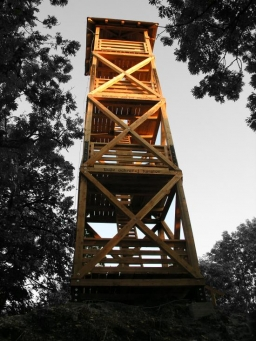Did you find an error or inaccuracy? Feel free to write us. Thank you!Tips to related online calculators
Need help to calculate sum, simplify or multiply fractions? Try our fraction calculator.
Check out our ratio calculator.
Do you want to convert length units?

#### You need to know the following knowledge to solve this word math problem:

We encourage you to watch this tutorial video on this math problem:

## Related math problems and questions:

• Vertical rodThe vertical one-meter-long rod casts a shadow 150 cm long. Calculate the height of a column whose shadow is 36 m long at the same time.The nine-meter poplar casts a shadow 16.2 m long. How long does a shadow cast by Peter at the same time, if it is 1.4 m high?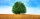The shadow of a 1 m high pole thrown on a horizontal plane is 0.8 m long. At the same time, the shadow of a tree thrown on a horizontal plane is 6.4 m. Determine the height of the tree.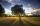Nine meters height poplar tree has a shadow 16.2 meters long. How long shadow have at the same time Joe if he is 1,4m tall?
• Chimney and treeCalculate the height of the factory chimney, which casts a shadow 6.5 m long in the afternoon. At the same time, a 6 m high tree standing near it casts a shadow 25 dm long.A 2-meter rod casts a shadow 3.2 m long. How high is a tree with a shadow of 14.4 m?
• There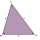There is a stretched steel cable between the 3 columns. The height of the first column is 4 m, the height of the second is 3.5 m. The distance between the first two columns is 2.5 m, the distance between the second and third is 5 m. The heels of all three
• Sun raysIf the sun's rays are at an angle 60° then famous Great Pyramid of Egypt (which is now high 137.3 meters) has 79.3 m long shadow. Calculate current height of neighboring chefren pyramid whose shadow is measured at the same time 78.8 m and the current heigMiro stands under a tree and watching its shadow and shadow of the tree. Miro is 180 cm tall and its shade is 1.5 m long. The shadow of the tree is three times as long as Miro's shadow. How tall is the tree in meters?The shadow of the tree is 16 meters long. Shadow of two meters high tourist sign beside standing is 3.2 meters long. What height has tree (in meters)?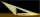A meter pole perpendicular to the ground throws a shadow of 40 cm long, the house throws a shadow 6 meters long. What is the height of the house?A boy of height 1.7m is standing 30m away from the flagstaff on the same level ground. He observes that the angle of deviation of the top of flagstaff is 30 degrees. Calculate the height of flagstaff.Mast has 13 m long shadow on a slope rising from the mast foot in the direction of the shadow angle at angle 15°. Determine the height of the mast, if the sun above the horizon is at angle 33°. Use the law of sines.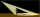The chimney casts a shadow 45 meters long. The one-meter-long rod standing perpendicular to the ground has a shadow 90 cm long. Calculate the height of the chimney.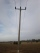On a flat plain, 2 columns are erected vertically upwards. One is 7 m high and the other 4 m. Cables are stretched between the top of one column and the foot of the other column. At what height will the cables cross? Assume that the cables do not sag.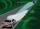Under traffic regulations, car lights can illuminate the road up to a maximum of 30 m. To check the reach of the dipped-beam lights of their car, Peter stopped the car at 1.5 m from the wall. The dipped beam headlights are 60 cm high. At what height on th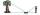How far must Paul place a mirror to see the top of the tower 12 m high? The height of Paul's eyes above the horizontal plane is 160 cm, and Paul is from the tower distant 20 m.